# JP2012003612A - Design support program, design support device and design support method - Google Patents

Design support program, design support device and design support method Download PDF

## Info

Publication number
JP2012003612A
JP2012003612A JP2010139637A JP2010139637A JP2012003612A JP 2012003612 A JP2012003612 A JP 2012003612A JP 2010139637 A JP2010139637 A JP 2010139637A JP 2010139637 A JP2010139637 A JP 2010139637A JP 2012003612 A JP2012003612 A JP 2012003612A
Authority
JP
Japan
Prior art keywords
elapsed time
cell
output
step
value
Prior art date
Legal status (The legal status is an assumption and is not a legal conclusion. Google has not performed a legal analysis and makes no representation as to the accuracy of the status listed.)
Granted
Application number
JP2010139637A
Other languages
Japanese (ja)
Other versions
JP5531807B2 (en
Inventor
Mitsuru Onodera

Original Assignee
Fujitsu Semiconductor Ltd

Priority date (The priority date is an assumption and is not a legal conclusion. Google has not performed a legal analysis and makes no representation as to the accuracy of the date listed.)
Filing date
Publication date
Application filed by Fujitsu Semiconductor Ltd, 富士通セミコンダクター株式会社 filed Critical Fujitsu Semiconductor Ltd
Priority to JP2010139637A priority Critical patent/JP5531807B2/en
Publication of JP2012003612A publication Critical patent/JP2012003612A/en
Application granted granted Critical
Publication of JP5531807B2 publication Critical patent/JP5531807B2/en
Expired - Fee Related legal-status Critical Current
Anticipated expiration legal-status Critical

• 238000004364 calculation methods Methods 0.000 claims description 88
• 238000000605 extraction Methods 0.000 claims description 15
• 238000000034 methods Methods 0.000 description 25
• 230000002123 temporal effects Effects 0.000 description 4

## Images

•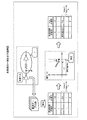•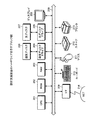•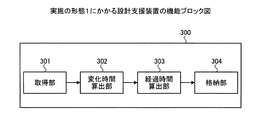•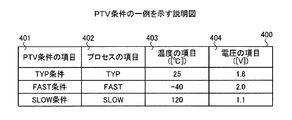•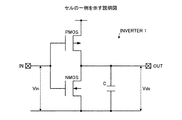•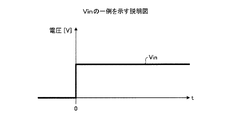•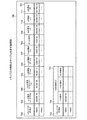•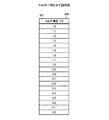•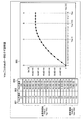••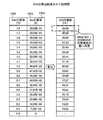•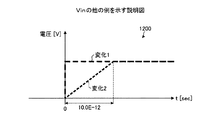•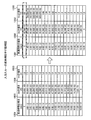••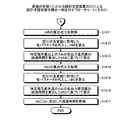••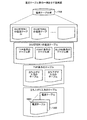••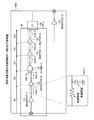••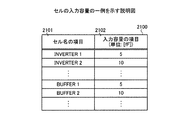•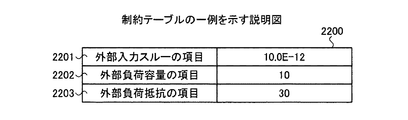•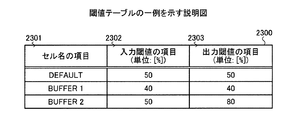•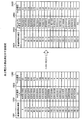•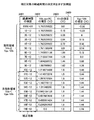••••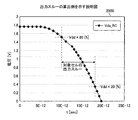•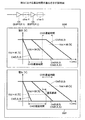•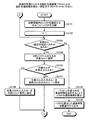•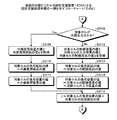•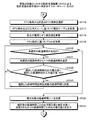•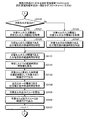## Classifications

• GPHYSICS
• G06COMPUTING; CALCULATING; COUNTING
• G06FELECTRIC DIGITAL DATA PROCESSING
• G06F30/30Circuit design
• G06F30/32Circuit design at the digital level
• G06F30/33Design verification, e.g. functional simulation or model checking
• G06F30/3308Design verification, e.g. functional simulation or model checking using simulation
• G06F30/3312Timing analysis

## Abstract

An output voltage that is dull in RC is reproduced to suppress a delay error.
A current table group stores, for each cell, an output voltage value of a cell at each of a plurality of elapsed times from the start of a change in input voltage applied to the cell, and a memory accessible to a design support apparatus. Stored in the device. The design support device extracts an output voltage value at each elapsed time related to the target cell selected from the circuit information of the design target circuit based on the target cell. The design support apparatus determines the elapsed time of Vds where Vds (output voltage) <Vgs (input voltage) −Vth (gate threshold voltage) as the correction target elapsed time. The design support apparatus corrects the elapsed time by adding the time constant on the output side of the target cell to the elapsed time to be corrected. The design support apparatus outputs an output voltage value for each elapsed time after correction and an output voltage value for each elapsed time that has not been determined as a correction target.
[Selection] Figure 1

## Description

The present invention relates to a design support program, a design support apparatus, and a design support method for supporting the design of a semiconductor integrated circuit.

Conventionally, in analog circuit design, a technique for simulating analog characteristics using a simulator such as SPICE is known.

On the other hand, in designing a digital circuit, an accurate delay time can be obtained by performing a simulation using a simulator such as SPICE (for example, refer to Patent Document 1 below) (hereinafter referred to as “Prior Art 1”). . However, there is a problem that enormous time is required to perform all the cells in the design target circuit using SPICE as in the prior art 1.

Therefore, a technique is known in which a delay time and output through (time required for rising (or rising) of an output voltage output from a cell) is specified for each cell used in design using a simulator such as SPICE and stored as a library. Yes. The delay time of each cell in the circuit to be designed has been calculated by referring to the library (for example, refer to Patent Document 2 below) (hereinafter referred to as “Prior Art 2”).

Further, it is known that the delay time of the path in the circuit to be designed is calculated by adding the delay times of the cells constituting the path.

JP-T-2004-501438 JP-A-10-198720

However, in the prior art 2, the load resistance (for example, wiring resistance) and load capacity (wiring capacity and input capacity of the next stage cell) on the output side of each cell are replaced with a lumped constant capacity. In the prior art 2, the temporal change of the output voltage is calculated by SPICE by giving the temporal change of the input voltage to the circuit model.

Since the values of load resistance and load capacitance differ from cell to cell depending on the layout of the circuit to be designed, there is a problem that an error occurs in the delay time compared to the case of using the prior art 1. Furthermore, since the output voltage value is calculated only from the value of the lumped constant capacitance, the transient response due to R (load resistance) C (load capacitance) is linearly approximated, compared with the case of using the prior art 1. There was a problem that an error occurred in the delay time. Moreover, when the prior art 1 was used as mentioned above, there existed a problem which took enormous time.

In particular, when the conventional technique 2 is used in a miniaturized process in recent years, there is an error between the delay time obtained by the conventional technique 2 and the delay time obtained by the conventional technique 1 due to lower voltage, higher integration, and higher speed. Prominently appear. That is, in recent years, there has been a problem that the influence of the delay time error cannot be ignored.

According to one aspect of the present invention, from a storage device that stores, for each cell, an output voltage value of the cell at each of a plurality of elapsed times from the start of a change in input voltage applied to the cell, the circuit to be designed The output voltage value at each elapsed time related to the target cell selected from the circuit information is extracted based on the target cell, and the specific voltage value is calculated from the elapsed time of each output voltage value of the extracted target cell. The elapsed time to be corrected is determined based on the above, the time constant on the output side of the target cell is added to the determined elapsed time, and the output voltage value for each elapsed time after correction and not determined by the determination step A design support program, a design support apparatus, and a design support method for outputting an output voltage value for each elapsed time are provided.

According to the present design support program, the design support apparatus, and the design support method, it is possible to reproduce an output voltage that is dull in RC and to suppress a delay error.

It is explanatory drawing which shows an example of this invention. It is a block diagram which shows the hardware of a design support apparatus. 1 is a functional block diagram of a design support apparatus according to a first exemplary embodiment; It is explanatory drawing which shows an example of PTV conditions. It is explanatory drawing which shows an example of a cell. It is explanatory drawing which shows an example of Vin. It is explanatory drawing which shows the table of a transistor characteristic. It is explanatory drawing which shows an example of Vds. It is explanatory drawing which shows an example of Ids for every Vds. It is explanatory drawing which shows Ids for every elapsed time. It is explanatory drawing which shows the calculation result of rDS. It is explanatory drawing which shows the other example of Vin. It is explanatory drawing which shows the example of reflection of an input through. 6 is a flowchart (part 1) illustrating an example of a design support processing procedure performed by the design support apparatus 300 according to the first embodiment; 10 is a flowchart (part 2) illustrating an example of a design support processing procedure by the design support apparatus 300 according to the first embodiment; FIG. 4 is a functional block diagram of a design support apparatus according to a second exemplary embodiment. It is explanatory drawing which shows an example of a current table group. It is explanatory drawing which shows an example of an internal resistance table group. It is explanatory drawing which shows an example of the circuit information of a design object circuit. It is explanatory drawing which shows an example of a wiring table. It is explanatory drawing which shows an example of the input capacity of a cell. It is explanatory drawing which shows an example of a constraint table. It is explanatory drawing which shows an example of a threshold value table. It is explanatory drawing which shows the example of calculation of an output voltage. It is explanatory drawing which shows the example of determination of the elapsed time of correction | amendment object. It is explanatory drawing which shows the example of correction | amendment. It is explanatory drawing which shows a complementation result. It is explanatory drawing which shows the example of calculation of delay time. It is explanatory drawing which shows the example of calculation of an output through. 10 is an explanatory diagram illustrating calculation of a delay time in Example 2. FIG. 10 is a flowchart (part 1) illustrating an example of a design support processing procedure by the design support apparatus 1600 according to the second embodiment; 10 is a flowchart (part 2) illustrating an example of a design support processing procedure by the design support apparatus 1600 according to the second embodiment; 10 is a flowchart (part 3) illustrating an example of a design support processing procedure by the design support apparatus 1600 according to the second embodiment; 10 is a flowchart (part 4) illustrating an example of a design support processing procedure by the design support apparatus 1600 according to the second embodiment;

Exemplary embodiments of a design support program, a design support apparatus, and a design support method according to the present invention will be explained below in detail with reference to the accompanying drawings.

FIG. 1 is an explanatory diagram showing an example of the present invention. The current table group holds the output voltage value of each cell at each of a plurality of elapsed times from the start of the change in the input voltage applied to the cell, and is stored in a storage device accessible by the design support device. ing. The output voltage value of the cell is a value calculated without considering the load resistance value and load capacitance value on the output side of the cell.

First, the design support apparatus extracts an output voltage value at each elapsed time related to the target cell selected from the circuit information of the design target circuit based on the target cell. Next, the design support apparatus determines the elapsed time of Vds where Vds (output voltage) <Vgs (input voltage) −Vth (gate threshold voltage) as the correction target elapsed time. In this embodiment, it is assumed that Vin is the same as Vgs.

Then, the design support apparatus corrects the elapsed time by adding the time constant on the output side of the target cell to the elapsed time to be corrected. The time constant is a value calculated based on the load resistance value and the load capacity value on the output side of the target cell. Next, the design support apparatus outputs an output voltage value for each elapsed time after correction and an output voltage value for each elapsed time that has not been determined as a correction target.

First, in the first embodiment, an example of creating a cell current table used in a circuit to be designed will be described. In the second embodiment, an example of calculating the delay time of the target cell selected from the circuit information of the circuit to be designed using the current table created in the first embodiment will be described.

(Embodiment 1)
(Hardware of design support equipment)
FIG. 2 is a block diagram illustrating hardware of the design support apparatus. In FIG. 2, the design support apparatus includes a CPU (Central Processing Unit) 201, a ROM (Read-Only Memory) 202, and a RAM (Random Access Memory) 203. The design support apparatus further includes a magnetic disk drive 204, a magnetic disk 205, an optical disk drive 206, an optical disk 207, a display 208, an I / F (Interface) 209, and a keyboard 210. The design support apparatus further includes a mouse 211, a scanner 212, and a printer 213. Each unit is connected by a bus 200.

Here, the CPU 201 governs overall control of the design support apparatus. The ROM 202 stores a program such as a boot program. The RAM 203 is used as a work area for the CPU 201. The magnetic disk drive 204 controls reading / writing of data with respect to the magnetic disk 205 according to the control of the CPU 201. The magnetic disk 205 stores data written under the control of the magnetic disk drive 204.

The optical disk drive 206 controls reading / writing of data with respect to the optical disk 207 according to the control of the CPU 201. The optical disk 207 stores data written under the control of the optical disk drive 206, or causes the computer to read data stored on the optical disk 207.

The display 208 displays data such as a document, an image, and function information as well as a cursor, an icon, or a tool box. As the display 208, for example, a CRT, a TFT liquid crystal display, a plasma display, or the like can be adopted.

The I / F 209 is connected to a network 214 such as a LAN (Local Area Network), a WAN (Wide Area Network), and the Internet through a communication line, and is connected to other devices via the network 214. The I / F 209 controls an internal interface with the network 214 and controls data input / output from an external device. For example, a modem or a LAN adapter can be adopted as the I / F 209.

The keyboard 210 includes keys for inputting characters, numbers, various instructions, and the like, and inputs data. Moreover, a touch panel type input pad or a numeric keypad may be used. The mouse 211 performs cursor movement, range selection, window movement, size change, and the like. A trackball or a joystick may be used as long as they have the same function as a pointing device.

The scanner 212 optically reads an image and takes in the image data into the design support apparatus. The scanner 212 may have an OCR (Optical Character Reader) function. The printer 213 prints image data and document data. As the printer 213, for example, a laser printer or an ink jet printer can be employed.

(Functional block diagram of the design support apparatus according to the first embodiment)
FIG. 3 is a functional block diagram of the design support apparatus according to the first embodiment. The design support apparatus 300 includes an acquisition unit 301, a change time calculation unit 302, an elapsed time calculation unit 303, and a storage unit 304. The CPU 201 loads a design support program having each function (acquisition unit 301 to storage unit 304) stored in a storage device such as the ROM 202, the RAM 203, the magnetic disk 205, and the optical disk 207 shown in FIG. And each function is implement | achieved when CPU201 performs the process coded by this design support program.

The acquisition unit 301 acquires the output current value at each output voltage value from a storage device that stores the output current value of the cell at each output voltage value of the plurality of output voltage values of the cell that are equal to or higher than the specific voltage value. Specifically, the specific voltage value is, for example, Vgs (Vin) −Vth.

The change time calculation unit 302 has a plurality of output voltage values based on the junction capacitance value of the transistor in the cell, the gate threshold voltage value (Vth) of the cell, and the output current value acquired by the acquisition unit 301. The change time during which the output current value changes from the lowest voltage value to the highest voltage value among the plurality of output voltage values is calculated.

Based on the change time calculated by the change time calculation unit 302, the elapsed time calculation unit 303 calculates the elapsed time from the start of the change in the input voltage to the cell for each output current value.

The storage unit 304 stores the elapsed time of each output current value calculated by the elapsed time calculation unit 303 in the storage device.

In addition, the acquisition unit 301 acquires the output current value at each output voltage value from the storage device that stores the output current value of the cell at each output voltage value of the plurality of output voltage values of the cell that is less than the specific voltage value. . Specifically, the specific voltage value is, for example, Vgs (Vin) −Vth.

Based on the value of the junction capacitance of the transistor in the cell, the value of the input voltage to the cell, and the output current value acquired by the acquisition unit 301, the change time calculation unit 302 is the most of the plurality of output voltage values. A change time during which the output current value changes from the low voltage value to the highest voltage value among the plurality of output voltage values is calculated.

Based on the change time calculated by the change time calculation unit 302, the elapsed time calculation unit 303 calculates the elapsed time from the start of input voltage change for each output current value.

The storage unit 304 stores the elapsed time of each output current value calculated by the elapsed time calculation unit 303 in the storage device.

Based on the above, the creation of an inverter current table will be described in detail as an example.

(PTV conditions)
FIG. 4 is an explanatory diagram showing an example of the PTV condition. First, PTV indicates a process, a temperature (temperature), and a voltage (voltage). The PTV condition table 400 includes a PTV condition item 401, a process item 402, a temperature item 403, and a voltage item 404. The item 401 of the PTV condition holds whether it is a TYP condition, a FAST condition, or a SLOW condition.

Each condition is as follows.
-TYP condition: A condition in which the operation of each cell is normal speed-FAST condition: A condition in which the operation of each cell is the fastest-SLOW condition: A condition in which the operation of each cell is the slowest

First, in the TYP condition, the process item 402 is the TYP condition (standard condition), the temperature item 403 is 25 ° C. (normal temperature), and the voltage item 404 is 1.8 [V]. Next, in the FAST condition, the process item 402 is the FAST condition (fast condition), the temperature item 403 is −40 ° C. (low temperature), and the voltage item 404 is 2.0V. Under the SLOW condition, the process item 402 is the SLOW condition (slow condition), the temperature item 403 is 120 [° C.] (high temperature), and the voltage item 404 is 1.1 [V]. The PTV condition table 400 is stored in a storage device such as the RAM 203, the magnetic disk 205, and the optical disk 207. The numerical values and PTV conditions in the PTV condition table 400 are examples.

(Example cell)
FIG. 5 is an explanatory diagram showing an example of a cell. In the first embodiment, an inverter cell will be described as an example. In FIG. 5, the cell of the inverter whose cell name is INVERTER1 is shown in the transistor description. The INVERTER 1 includes a PMOS (P Channel Metal Oxide Semiconductor) and an NMOS (N Channel Metal Oxide Semiconductor). The time difference between the input voltage (Vin) input to the IN terminal and the output voltage (Vds) output from the OUT terminal is the delay time. C shown in FIG. 5 is a junction capacitance (parasitic capacitance) in INVERTER1, which is different from the load capacitance on the output side of INVERTER1.

Here, Vds, Vds_nonRC, and Vds_RC used in the first and second embodiments will be described. Vds, Vds_nonRC, and Vds_RC all indicate output voltages output from the OUT terminal. Vds used in the first embodiment is an output voltage set by the user. Vds_nonRC used in the second embodiment is an output voltage calculated from the current table created in the first embodiment. Vds_RC used in the second embodiment is an output voltage obtained by correcting Vds_nonRC based on the load resistance and the load capacitance.

In the first embodiment, a case where a rising change input is given to the IN terminal will be described as an example. Since the rise delay time and the fall delay time are symmetric, the description of an example in the case where the fall change input is given to the IN terminal will be omitted. In the first embodiment, the output current (Ids) of the OUT terminal when a rising change input is given to the IN terminal is calculated, and the calculated result is tabulated.

FIG. 6 is an explanatory diagram showing an example of Vin. In the first and second embodiments, the change start of Vin is set to t = 0. In the first embodiment, Ids is obtained for each elapsed time from the start of the change. Further, if Vin = 0.0 [V] before t = 0, Vds = Vdd, and when the input of Vin rises at t = 0, the PMOS is immediately cut off. Therefore, here, only the NMOS will be described.

Here, as is well known, the saturation region and the non-saturation region which are the operation regions of the MOS are classified as follows.
Saturation region: Vds ≧ Vgs−Vth
Unsaturated region (linear region): Vds <Vgs−Vth

Then, the calculation formula (1) of the MOS output current (Ids) when the MOS operation region is the saturation region, and the calculation formula (2) of the MOS output current (Ids) when the MOS operation region is the non-saturation region (2) ) And are shown below.

The above equations (1) and (2) are stored in a storage device such as the RAM 203, the magnetic disk 205, and the optical disk 207. Each parameter is as follows.
[Mu] [cm ^ 2 / (V * s)]: carrier mobility [epsilon] 0 (Epsilon0) [F / cm]: vacuum dielectric constant [epsilon] ox (Epsilon ox) [F / cm]: relative dielectric constant [Tox [m] ]: Oxide film pressure • Cox [F]: Gate capacitance • W [m]: Gate width • L [m]: Gate length • C [F]: Junction capacitance (parasitic capacitance)

(Table of transistor characteristics)
FIG. 7 is an explanatory diagram showing a table of transistor characteristics. The transistor characteristic table 700 includes a table 701 and a table 712. The transistor characteristic table 700 is stored in a storage device such as the magnetic disk 205 or the optical disk 207.

The table 701 stores parameters for each MOS in INVERTER1. Table 701 includes transistor item 702, μ item 703, Cox item 704, ε0 item 705, εox item 706, Tox item 707, W / L item 708, and W It has an item 709, an L item 710, and a C item 711.

The transistor item 702 holds the type of MOS in INVERTER1. The μ item 703 holds μ, the Cox item 704 holds Cox, the ε0 item 705 holds ε0, and the εox item 706 holds εox. The Tox item 707 holds Tox, the W item 709 holds W, the L item 710 holds L, and the C item 711 holds C.

Further, the W / L item 708 holds the ratio of W held in the W item 709 and L held in the L item 710. For example, 35.42E-06 held in the Cox item 704 indicates 35.42 × 10 ^ (− 6). “^” Is a multiplier.

The table 712 includes a transistor item 713 and a Vth item 714. The transistor item 713 holds the type of MOS in INVERTER1. The Vth item 714 stores Vth (gate threshold voltage) of each transistor in the INVERTER 1 for each PTV condition.

Specifically, for example, the CPU 201 selects a specific PTV condition from the PTV condition table 400. Alternatively, for example, the CPU 201 sequentially selects PTV conditions from the PTV condition table 400. In the first embodiment, the TYP condition will be described as an example. That is, Vdd is 1.8 [V].

(Vds)
FIG. 8 is an explanatory diagram illustrating an example of Vds. The table 800 includes a Vds item 801, and the Vds item 801 holds Vds. In the first embodiment, as shown in FIG. 8, Vds is changed from 1.8 [V] to 0.0 [V] in 0.1 [V] steps, and the above formula (1) or the above formula (2) is used. To calculate Ids. When Vds is 1.8 to 1.5 [V], the operation region of INVERTER1 is a saturation region, and therefore Ids is calculated by the above equation (1). When Vds is 1.4 to 0.0 [V], the operation region of INVERTER1 is a non-saturation region, so Ids is calculated by the above equation (2).

<Calculation of Ids>
Specifically, for example, the CPU 201 acquires the above equation (1) from the storage device, acquires each parameter in the equation (1) from the transistor characteristic table 700, and sets it in the above equation (1). For example, the CPU 201 calculates Ids when the NMOS operation region is in the saturation region. That is, Ids is calculated when Vds is 1.8 to 1.5 [V]. In the saturation region, Ids has the same current value regardless of Vds. The calculation result is stored in a storage device such as the RAM 203, the magnetic disk 205, and the optical disk 207.

Specifically, for example, the CPU 201 acquires the above formula (2) from the storage device, acquires the parameters in the above formula (2) from the transistor characteristic table 700, and sets them in the above formula (2). . For example, the CPU 201 obtains Vds (1.4 to 0.0 [V]) from the table 800 in the order of steps, sets it to the above equation (2), and sets Ids in the non-saturated region to Vds (1.4 to 0). .0 [V]). The calculation result is stored in a storage device such as the RAM 203, the magnetic disk 205, and the optical disk 207.

<Ids for each elapsed time>
FIG. 9 is an explanatory diagram illustrating an example of Ids for each Vds. The table 900 indicates Ids for each Vds, and includes a Vds item 901 and an Ids item 902. The Vds item 901 holds Vds, and the Ids item 902 holds the Ids of the Vds.

In the graph 903, the horizontal axis is Vds [V], and the vertical axis is Ids [A]. In the graph 903, the current value is high when Vds is high, and the current value is low when Vds is low. Here, for example, Vds = Vgs is point A, Vds = Vgs−Vth is point B, Vgs / 2 is point C, and Vds = 0 is point D.

In the first embodiment, the time taken for the change in the output current of INVERTER1 from point A to point B is tAB, and the time taken for the change to change from point B to point C is tBC. The time taken for the change to change from point C to point D is defined as tCD. tAB is calculated from the above equation (1) by using the following equation (3).

Next, tBC will be described. Since the time integration of the current is the charge amount, the following formula (4) is obtained from the calculation formula of Ids in the non-saturation region.

Since the above equation (5) is derived from the above equation (4), when the left side of the above equation (5) is integrated from 0 to tBC and the right side is integrated from Vgs−Vth to 1/2 × Vgs, the following equation (6) is obtained. can get.

Expressions (3) and (6) are stored in a storage device such as the RAM 203, the magnetic disk 205, and the optical disk 207.

First, specifically, for example, the CPU 201 acquires the above expression (3) from the storage device by the change time calculation unit 302. Then, for example, the CPU obtains each parameter in the obtained equation (3) from the transistor characteristic table 700, sets it in the above equation (3), and calculates tAB. Next, specifically, for example, the CPU 201 acquires the above equation (6) from the storage device by the change time calculation unit 302. Then, for example, the CPU obtains each parameter in the obtained equation (6) from the transistor characteristic table 700, sets it in the above equation (6), and calculates tBC.

The calculation results are shown below.
TAB: 3.279355511146584E-12
TBC: 5.17154478518248E-12

Next, for example, based on tAB and tBC calculated by the CPU 201, Ids for each Vds is converted into Ids for each elapsed time.

FIG. 10 is an explanatory diagram showing Ids for each elapsed time. Here, the time from the start of the change of Vin is defined as the elapsed time. Here, since tAB is a change time during which the output current value changes from 1.8 to 1.5 [V], the CPU 201 uses, for example, the elapsed time calculation unit 303 to change the output current value at each Vds (or each Ids). The elapsed time is calculated as follows.

-Elapsed time [sec] when Vds is 1.8 [V]: 0
Elapsed time [sec] when Vds is 1.7 [V]: tAB / 3
Elapsed time [sec] when Vds is 1.6 [V]: tAB / 3 + (elapsed time when 1.7 [V])
Elapsed time [sec] when Vds is 1.5 [V]: tAB / 3 + (elapsed time when 1.6 [V])

Since tBC is a change time in which the output current value changes from 1.4 [V] to 0.9 [V], the CPU 201 calculates the elapsed time at each Vds (or each Ids) as follows, for example. calculate.

Elapsed time [sec] when Vds is 1.4 [V]: tBC / 6 + (elapsed time when 1.5 [V])
Elapsed time [sec] when Vds is 1.3 [V]: tBC / 6 + (elapsed time when 1.4 [V])
Elapsed time [sec] when Vds is 1.2 [V]: tBC / 6 + (elapsed time when 1.3 [V])
Elapsed time [sec] when Vds is 1.1 [V]: tBC / 6 + (elapsed time when 1.2 [V])
Elapsed time [sec] when Vds is 1.0 [V]: tBC / 6 + (elapsed time when 1.1 [V])
Elapsed time [sec] when Vds is 0.9 [V]: tBC / 6 + (elapsed time when 1.0 [V])

An example of Ids for each elapsed time is the table 1000. The table 1000 includes an elapsed time item 1001 and an Ids item 1002. In the table 1000, the elapsed time is held for each Ids at 1.8 [V] to 0.9 [V].

Further, when the resolution (TIMESCALE) is 1.0E-12 [sec], an example of the elapsed time of each Ids is the current table 1003. The current table 1003 has an elapsed time item 1004, an Ids item 1005, and a Vin item 1006. The Vin item 1006 is omitted in FIG.

The input threshold and the output threshold at the time of delay time calculation are Vdd × 50 [%], and the points C to D are Vds (when Vdd = Vds) × 50 [%] or less. Therefore, in the current table 1003, TIMESCALE is assigned from the point 900 to the point D along the steps of Vds from the table 900. Not limited to this, since the operation region from point C to point D is a non-saturated region, for example, the user creates a calculation formula in advance in the same manner as the calculation from point B to point C, and calculates tCD. May be. Further, the CPU 201 causes the storage unit 304 to store the current table 1003 in a storage device such as the RAM 203, the magnetic disk 205, and the optical disk 207.

<Calculation of rDS>
Next, calculation of the internal resistance (rDS) of the NMOS in INVERTER1 will be described. Specifically, for example, the CPU 201 calculates rDS by dividing Vds by Ids.

FIG. 11 is an explanatory diagram showing the calculation result of rDS. For example, the CPU 201 calculates the rDS for each Vds by dividing the Ids for which the Vds is calculated for each Vds. Here, rDS when Vds = 1.8 [V] is determined as the value of the internal resistance of the NMOS of INVERTER1. The determined rDS is stored in a storage device such as the RAM 203, the magnetic disk 205, and the optical disk 207.

<Tr and tf>
Next, creation of a current table in consideration of the influence of tr and tf will be described. Here, tr represents the time until the rising input voltage (Vin) rises, and tf represents the time until the falling input voltage (Vin) falls.

FIG. 12 is an explanatory diagram showing another example of Vin. A graph 1200 shows a change example of two Vins of change 1 and change 2. Change 1 is Vin that has risen without delay in the same manner as Vin shown in FIG. That is, in the case of change 1, tr is 0. On the other hand, change 2 takes 10E-12 [sec] from 0.0 [V] to Vdd [V]. That is, in the case of change 2, tr is 10E-12 [sec]. When the tr is reflected in the current table 1003, the result is as follows.

FIG. 13 is an explanatory diagram illustrating an example of reflection of input through. Here, for example, the delay time depending on the input slew is tr up to Vth. That is, 10E−12 / 1.8 × 0.36 (tr / Vdd × Vth) = 2.0E−12 [sec] is an input slew-dependent delay time. Specifically, for example, the CPU 201 can create the current table 1300 by adding a delay time of 2.0E-12 to the current table 1003.

The current table 1300 includes an elapsed time item 1301, an Ids item 1302, and a Vin item 1303. An elapsed time item 1301 holds the elapsed time, an Ids item 1302 holds Ids, and a Vin item 1303 holds Vin. Further, the value of the Vin item 1303 is set so that the elapsed time becomes 1.8 [V] at 10E-12 [sec].

Next, for example, the CPU 201 outputs a current table 1003 and a current table 1300. Examples of the output format include display on the display 208, print output to the printer 213, and transmission to an external device via the I / F 209. Alternatively, the data may be stored in a storage device such as the RAM 203, the magnetic disk 205, and the optical disk 207.

(Design support processing procedure of the design support apparatus 300 according to the first embodiment)
14 and 15 are flowcharts illustrating an example of a design support processing procedure by the design support apparatus 300 according to the first embodiment. First, the CPU 201 acquires each parameter of the transistor (step S1401). Then, the CPU 201 selects a specific PTV condition from a plurality of PTV conditions (step S1402), and acquires Vth of the selected PTV condition (step S1403).

Then, the CPU 201 acquires a plurality of Vds of the selected PTV condition (step S1404), and acquires the Ids calculation formula (1) related to the saturation region (step S1405). CPU201 substitutes each parameter acquired for each variable of a formula (1), and calculates Ids for every Vds more than a specific voltage value among a plurality of Vds (Step S1406). As described above, the specific voltage value is Vgs (Vin) −Vth.

Next, the CPU 201 obtains an Ids calculation formula (2) regarding the non-saturated region (step S1407). CPU201 substitutes each parameter acquired for each variable of a formula (2), and calculates Ids for every Vds below a specific voltage value among a plurality of Vds (Step S1408). Then, the CPU 201 calculates an internal resistance value based on Ids and Vds (step S1409), and outputs the internal resistance value (step S1410).

Next, the CPU 201 acquires the calculation formula (3) of tAB (step S1411), substitutes the acquired parameters for each variable of the formula (3), and calculates tAB (step S1412). And CPU201 calculates based on tAB which calculated the elapsed time of each output current value of Vds more than a specific voltage value (step S1413).

Then, the CPU 201 acquires the calculation formula (6) of tBC (step S1414), substitutes the acquired parameters for each variable of the formula (6), and calculates tBC (step S1415). Then, the CPU 201 calculates the elapsed time of each output current value of Vds less than the specific voltage value based on tBC (step S1416).

Then, the CPU 201 stores the elapsed time calculated for each Ids (step S1417), and the series of processing ends.

(Embodiment 2)
Next, in the second embodiment, an example in which the delay time of the target cell in the circuit information of the design target circuit is calculated using the current table created in the first embodiment will be described. In the second embodiment, since the hardware described in the first embodiment is the same, the same reference numerals are used and the description thereof is omitted.

(Functional block diagram of the design support apparatus according to the second embodiment)
FIG. 16 is a functional block diagram of the design support apparatus according to the second embodiment. The design support apparatus 1600 includes an extraction unit 1601, a determination unit 1602, a correction unit 1603, an output unit 1604, a specification unit 1605, and a calculation unit 1606. The CPU 201 loads a design support program having each function (extraction unit 1601 to calculation unit 1606) stored in a storage device such as the ROM 202, the RAM 203, the magnetic disk 205, and the optical disk 207 shown in FIG. And each function is implement | achieved when CPU201 performs the process coded by this design support program.

The extraction unit 1601 stores the output voltage value of the cell at each of a plurality of elapsed times from the start of the change in the input voltage applied to the cell, from the circuit information of the circuit to be designed, from the storage device. The output voltage value at each elapsed time relating to the selected target cell is extracted based on the target cell.

The determination unit 1602 determines the elapsed time to be corrected based on the specific voltage value from the elapsed time of each output voltage value extracted by the extraction unit 1601. The specific voltage value is Vgs (Vin) −Vth.

The correction unit 1603 adds the time constant on the output side of the target cell to the elapsed time determined by the determination unit 1602.

The output unit 1604 outputs an output voltage value for each elapsed time after correction by the correction unit 1603 and an output voltage value for each elapsed time not determined by the determination unit 1602.

The specifying unit 1605 specifies the elapsed time of the output voltage value that is the threshold value of the output voltage of the target cell among the output voltage values output by the output unit 1604.

The calculation unit 1606 calculates the time difference between the elapsed time from the start of the change in the input voltage to the target cell until the input voltage becomes the threshold value of the input voltage, and the elapsed time specified by the specifying unit 1605.

Further, the specifying unit 1605 specifies the elapsed time of the output voltage value that is the threshold value of the input voltage of the next-stage cell of the target cell among the output voltage values output by the output unit 1604.

The calculation unit 1606 calculates the time difference between the elapsed time from the start of the change in the input voltage to the target cell until the input voltage becomes the threshold value of the input voltage, and the elapsed time specified by the specifying unit 1605.

This will be described in detail based on the above.

(Current table group)
FIG. 17 is an explanatory diagram illustrating an example of a current table group. The current table group 1700 has a current table for each cell. Taking INVERTER1 as an example, the current table group 1700 has a current table for each cell, such as an INVERTER1 current table and an INVERTER2 current table. The INVERTE1 current table group includes a TYP condition table group, a FAST condition table group, and a SLOW condition table group. Further, the table group of the TYP condition includes a rising input table and a falling input table.

Further, the falling input table has a table for each tf, and the rising input table has a table for each tr. The rising input table group includes, for example, the current table 1003 and the current table 1300 shown in the first embodiment. The current table group 1700 is stored in a storage device such as the RAM 203, the magnetic disk 205, and the optical disk 207, for example.

(Internal resistance table)
FIG. 18 is an explanatory diagram showing an example of the internal resistance table group. The internal resistance table group 1800 holds the value of the internal resistance of the MOS in each cell for each cell. For example, the internal resistance table 1801 holds the value of the NMOS internal resistance of INVERTER1 and the value of the PMOS internal resistance of INVERTER1.

In the second embodiment, a table having an output voltage value for each elapsed time of the target cell selected from the circuit information of the design target circuit is calculated based on the table 1300 having the output current value for each elapsed time and the internal resistance table 1801. is doing. However, the present invention is not limited to this, and the user may create a table having output voltage values for each elapsed time in advance for each cell.

(Circuit information of the design target circuit)
FIG. 19 is an explanatory diagram of an example of circuit information of a design target circuit. The circuit information 1900 is electronic data including cell connection information in the circuit to be designed, and specifically, for example, layout data of the circuit to be designed and a netlist of the circuit to be designed after logic synthesis. The circuit information 1900 includes cells C1 to C7.

Here, the instance name is a unique name of each cell in the circuit to be designed, and the cell name is a name for indicating the type of cell. The cells C1 to C7 are instance names, and INVERTER1, INVERTER2, 2AND1 (two inputs and one output AND), BUFFER1, BUFFER2, and FF1 are cell names.

For example, both inverters such as INVERTER1 and INVERTER2 are inverters, and even if the logic is the same, the performance is different. For example, the cell C1 and the cell C7 have different instance names, but both are INVERTER1. In the second embodiment, even if cells C1 and C7 have the same cell name, the wiring on the output side of each cell and the next cell of the cell are different, so the time constants are different and the previous cell of the cell is different. So the input through is different. Therefore, different delay times are calculated for the cell C1 and the cell C7.

For example, the CPU 201 calculates the delay time of each cell from the cells C1 to C5 on the path P, and sums the calculated delay times, whereby the delay time of the path P can be calculated. Therefore, for example, the CPU 201 divides the path P for each cell and calculates delay times of the divided paths as paths P1 to P5.

Here, the path is a set of a series of cells and nets from an input terminal to a macro (for example, FF (flip flop)), and a set of cells and nets between macros (for example, FF (flip flop)). is there. Alternatively, a path is a set of a series of cells and nets from a macro to an output terminal.

Further, the output-side wiring of the cell C1 is line1, the output-side wiring of the cell C2 is line2, and the output-side wiring of the cell C3 is line3. The wiring on the output side of the cell C4 is line4, and the wiring on the output side of the cell C5 is line5. In the semiconductor integrated circuit, since the wiring is formed of METAL or Polysilicon, the wiring is represented by resistance (wiring resistance) or capacitance (wiring capacitance) as indicated by line 1 in FIG.

(Wiring table)
FIG. 20 is an explanatory diagram illustrating an example of a wiring table. First, the wiring table 2000 holds the wiring resistance value and wiring capacitance value of the wiring from the output of each cell to the input to the next cell in the circuit information 1900. The wiring table 2000 includes a wiring item 2001, a wiring resistance item 2002, and a wiring capacitance item 2003.

The wiring item 2001 holds a wiring name, the wiring resistance item 2002 holds a wiring resistance value, and the wiring capacitance item 2003 holds a wiring capacitance value. Note that the wiring table 2000 is created by a designer based on wiring information extracted from layout data of a circuit to be designed, for example.

Returning to the description of FIG. 19, for example, the next cell of the cell C1 is the cell C2. The input capacity of the cell C2 becomes a part of the load capacity on the output side of the cell C1 (the load capacity of the cell C1). Here, the input capacitance of the cell is, for example, the gate capacitance of the MOS in the cell.

(Cell input capacity)
FIG. 21 is an explanatory diagram showing an example of the input capacity of a cell. The input capacity table 2100 holds the input capacity value of each cell. The input capacity table 2100 has a cell name item 2101 and an input capacity item 2102. For example, when the cell name held in the cell name item 2101 is INVERTER1, the input capacity item 2100 holds 5 [fF].

Returning to the description of FIG. 19, for example, there is no next stage cell of the cell C7, and the next stage of the cell C7 is an output terminal. In this case, since the load resistance and load capacity on the output side of the cell C7 are unknown, in the second embodiment, the value of the external load resistance or the external load capacity defined in advance by the user (designer or verifier) is determined. The values are used for the load resistance value of the cell C7 and the load capacity value of the cell C7, respectively.

Further, there is no preceding cell of the cell C1, and the preceding stage of the cell C1 is an input terminal. In this case, since the input through is unknown, in the second embodiment, an external input through defined in advance by a user (designer or verifier) is used for the input through of the cell C1.

(Constraint table)
FIG. 22 is an explanatory diagram of an example of a constraint table. The constraint table 2200 stores an external input through, an external load capacitance value, and an external load resistance value. In the second embodiment, as described above, the value held in the external input through item 2201 in the constraint table 2200 is used for the input through of the cell C1. As described above, in the second embodiment, the load capacity and the load resistance of the cell C7 are held in the external load capacity item 2202 and the external load resistance item 2203 in the constraint table 2200, respectively. Value is used.

(Threshold table)
FIG. 23 is an explanatory diagram of an example of the threshold value table. In the threshold value table 2300, when the delay time is calculated based on the temporal change of the input voltage and the temporal change of the output voltage, the threshold value of the input voltage (hereinafter referred to as “input threshold value”) and the output voltage. A threshold value (hereinafter referred to as “output threshold value”) is held. The threshold table 2300 includes a cell name item 2301, an input threshold item 2302, and an output threshold item 2303.

A cell name item 2301 holds a cell name, an input threshold value item 2302 holds an input threshold value, and an output threshold value item 2303 holds an output threshold value. Taking the case where the cell name item 2301 is DEFAULT as an example, the input threshold is 50 [%] and the output threshold is 50 [%]. In this case, the time difference from the elapsed time when Vin is Vdd × 50 [%] to the elapsed time when Vds is Vdd × 50 [%] is the delay time of the target cell.

Next, a specific processing example will be described. First, in Example 1, calculation of delay time and input through will be described. Next, in Example 2, a case where the threshold value of the next cell of the target cell is different from the threshold value of the target cell will be described.

(Example 1)
<Calculation of time constant>
First, specifically, for example, the CPU 201 selects the cell C1 from the circuit information 1900 as a target cell. Next, specifically, for example, the CPU 201 determines the value of the input capacitance of the next cell (cell C2) of the cell C1, the value of the wiring capacity of the line 1 on the output side of the cell C1, and the value of the wiring resistance of the line 1. To get. Then, for example, the CPU 201 determines whether or not there is a preceding cell of the cell C1, and since there is no preceding cell, the CPU 201 obtains the value held in the external input through item 2201 in the constraint table 2200 and obtains the cell. C1 input through is determined.

The acquisition results are shown below.
The value of the input capacity of the next cell (cell C2) of the cell C1: 5 [fF]
-Line 1 wiring capacitance value: 5 [fF]
-Value of wiring resistance of line1: 50 [Ω]
・ External input through: 10E-12 [sec]

In the second embodiment, since the CPU 201 automatically selects the target cell, the CPU 201 accesses the storage device and acquires the wiring capacitance value, the wiring resistance value, and the external input through. For example, the user selects a target cell, and the user inputs the value of the wiring capacitance, the value of the wiring resistance, and the external input through to the design support apparatus 1600, and the CPU 201 May be accepted.

Next, specifically, for example, since the cell name of the cell C1 is INVERTER1, the CPU 201 uses the extraction unit 1601 to convert the current table of rising input or falling input from the INVERTER1 current table based on the external input through. Extract. Here, taking the rising input as an example, the current table 1300 is acquired.

Next, specifically, for example, the CPU 201 calculates the value of the load capacity of the cell C1 based on the value of the input capacity of the cell C2 and the value of the wiring capacity of the line1.
-Load capacitance value of cell C1 = input capacitance value of cell C2 + wiring capacitance value of line1
= 5.0E-15 [F] + 5.0E-15 [F]
= 10.0E-15 [F]

Then, for example, the CPU 201 calculates the time constant by multiplying the load capacitance value of the cell C1 by the wiring resistance value of the line1. The calculation results are shown below.
The time constant of the cell C1 = the load capacitance value of the cell C1 × the wiring resistance value of the line1
= 10.0E-15 [F] x 50 [Ω]
= 0.5E-12 [sec]

<Calculation of output voltage value>
FIG. 24 is an explanatory diagram illustrating an example of calculating the output voltage. Next, specifically, for example, the CPU 201 calculates the value of the output voltage for each elapsed time based on the extracted current table and the internal resistance of the NMOS of INVERTER1. A table 2400 is a calculation result. The table 2400 includes an elapsed time item 2401, a Vds_nonRC item 2402, and a Vin item 2403. The table 2400 is stored in a storage device such as the RAM 203, the magnetic disk 205, and the optical disk 207.

Next, the elapsed time of Vds_nonRC in which the operation region of INVERTER1 becomes the saturation region is not corrected by the time constant, and the elapsed time of Vds_nonRC in which the operation region of INVERTER1 becomes the non-saturation region is corrected by the time constant. In the second embodiment, when the operation region is the saturation region, the output current is sufficiently flowing, so that the influence of the load capacitance and load resistance of the cell C1 is small. Therefore, the elapsed time of Vds_nonRC where the operation region is the saturation region Is not corrected.

Specifically, for example, the CPU 201 causes the determination unit 1602 to calculate Vgs (Vin) −Vth for each Vds_nonRC. Then, for example, the CPU 201 determines whether or not Vds_nonRC <Vgs (Vin) −Vth for each Vds_nonRC by the determination unit 1602. For example, if Vds_nonRC <Vgs (Vin) −Vth is determined by the determining unit 1602, the CPU 201 determines the elapsed time of Vds_nonRC as the elapsed time to be corrected.

FIG. 25 is an explanatory diagram of an example of determining the elapsed time to be corrected. For example, when the elapsed time is 10E-12 [sec], Vds_nonRC is about 1.51 [V], and Vgs-Vth is 1.44 [V]. Therefore, since Vds_nonRC ≧ Vgs−Vth, when the elapsed time is 10E−12 [sec], the NMOS operation region of INVERTER1 is a saturation region.

Next, for example, when the elapsed time is 11E-12 [sec], Vds_nonRC is about 1.41 [V], and Vgs-Vth is 1.44 [V]. Therefore, since Vds_nonRC ≧ Vgs−Vth, when the elapsed time is 11E-12 [sec], the NMOS operating region of INVERTER1 is a non-saturated region. Therefore, the elapsed time 10E-12 is determined as a correction target. Here, 11E-12 to 19E-12 [sec] is determined as the elapsed time to be corrected.

FIG. 26 is an explanatory diagram illustrating a correction example. Specifically, for example, the CPU 201 adds the calculated time constant of the cell C1 to each elapsed time determined by the correction unit 1603 as the elapsed time to be corrected, thereby reducing the elapsed time of the correction target. to correct.

In FIG. 26, for example, t0 is not a correction target, but t1 and t2 are elapsed times that are correction targets. In the graph 2604, correction is performed by adding τ to t1 and t2. The correction results of t1 and t2 are t1 'and t2', respectively. Then, for example, the CPU 201 causes the output unit 1604 to output an output voltage value for each elapsed time after correction and an output voltage value for each elapsed time that has not been determined as a correction target.

Examples of the output format include display on the display 208, print output to the printer 213, and transmission to an external device via the I / F 209. Alternatively, the data may be stored in a storage device such as the RAM 203, the magnetic disk 205, and the optical disk 207.

An example of the output result is a table 2600. The table 2600 includes an elapsed time item 2601, a Vds_RC item 2602, and a Vin item 2603. The elapsed time item 2601 holds the elapsed time, the Vds_RC item 2602 holds Vds_RC, and the Vin item 2603 holds Vin. Note that the voltage value of Vds_RC is not changed from that of Vds_nonRC, but the elapsed time is corrected.

Furthermore, when the number of measurements (the number of elapsed times) is small, the plurality of elapsed times of the output voltage value for each elapsed time of the plurality of elapsed times may be supplemented. Specifically, for example, the CPU 201 calculates Vds_RC at an intermediate elapsed time (tZ) between one elapsed time (tX) and the next elapsed time (tY) after one elapsed time by the following equation (7). It complements by calculating using.

Vds_RC (tZ) = {Vds_RC (tX) [V] −Vds_RC (tY) [V]} / {tX [psec] −tY [psec]} × τ [psec] + Vds_RC (tY) [V]
... (7)

For example, the case where tA and tB are as follows is described as an example.
・ TX = 13.5 [psec]
・ TY = 12.5 [psec]
・ TZ = 13 [psec]
・ Vds (tX) = 1.162 [V]
・ Vds (tY) = 1.297 [V]
・ Τ = 0.5 [psec]
Vds_RC (13) = {1.297 [V] −1.162 [V]} / {13.5 [psec] −12.5 [psec]} × 0.5 [psec] +1.162 [V]
= 1.229 [V]

FIG. 27 is an explanatory diagram showing a complement result. The table 2700 is a complement result obtained by complementing the table 2700 using the above equation (7). The table 2700 includes an elapsed time item 2701, a Vds_RC item 2702, and a Vin item. In FIG. 27, the item Vin is omitted. As an example, the complement is performed only between 12.5 [psec] and 19.5 [psec].

<Calculation of delay time and output through>
Next, calculation of the delay time of the target cell will be described. Specifically, for example, the CPU 201 searches the threshold value table 2300 for the input threshold value of the target cell and the output threshold value of the target cell based on the cell name. Since there is no cell name corresponding to INVERTER1 in the threshold value table 2300, the threshold value of DEFAULT is searched. The input threshold value of DEFAULT is 50 [%], and the output threshold value of DEFAULT is 50 [%].

Specifically, for example, the CPU 201 uses the specifying unit 1605 to specify the elapsed time of Vds_RC that is the same as the output threshold value of the target cell acquired from the table 2700. Further, for example, the CPU 201 specifies the elapsed time of Vin that is the same as the input threshold value of the target cell acquired from the table 2700 by the specifying unit 1605. For example, the CPU 201 uses the calculation unit 1606 to calculate the time difference between the specified elapsed time of Vin and the specified elapsed time of Vds_RC as the delay time.

FIG. 28 is an explanatory diagram of an example of calculating the delay time. In the graph 2800, the input voltage value for each elapsed time is plotted, and a broken line (input voltage broken line) in which the plots are connected in the order of the elapsed time is shown. Further, in the graph 2800, the output voltage value for each elapsed time is plotted, and a broken line (output voltage broken line) in which the plots are connected in the order of the elapsed time is shown. In the graph 2800, the horizontal axis represents elapsed time, and the vertical axis represents voltage.

The input threshold is 50 [%], and the output threshold is 50 [%]. The time difference from the elapsed time when Vdd × 50 [%] of the input voltage broken line to the elapsed time when Vdd × 50 [%] of the output voltage broken line is the delay time of the target cell. Further, the input voltage line and the output voltage line are simply connected between the plots in the order of elapsed time. However, the present invention is not limited to this, and approximation such as linear approximation may be performed.

Next, calculation of output through of the target cell will be described. The output through of the target cell is used as the input through of the next cell of the target cell. For example, the output slew of the falling output of the target cell is used when calculating the delay time of the falling input of the next cell, and the output slew of the rising output of the target cell is the calculation of the delay time of the rising input of the next cell. Sometimes used.

In the second embodiment, in the case of the falling output, the output through is a time difference from the elapsed time at Vdd × 80 [%] to the elapsed time at Vdd × 20 [%]. In the second embodiment, in the case of rising output, the output through is a time difference from the elapsed time at Vdd × 20 [%] to the elapsed time at Vdd × 80 [%].

Specifically, for example, the CPU 201 uses the specifying unit 1605 to calculate the elapsed time of Vds_RC that is Vdd × 80 [%] from the table 2700 and the elapsed time of Vds_RC that is Vdd × 20 [%] from the table 2700. Identify. Next, for example, the CPU 201 uses the calculation unit 1606 to calculate the time difference between the two specified elapsed times as an output through.

FIG. 29 is an explanatory diagram of an example of calculation of output through. In the graph 2900, the output voltage value for each elapsed time is plotted, and a broken line in which the plots are connected in the order of the elapsed time is shown. In the graph 2900, the horizontal axis represents elapsed time, and the vertical axis represents voltage.

The time difference from the elapsed time of the broken line Vdd × 80 [%] to the elapsed time of Vdd × 20 [%] is the output through. Then, for example, the CPU 201 outputs the calculated delay time and output through. Examples of the output format include display on the display 208, print output to the printer 213, and transmission to an external device via the I / F 209. Further, the output format may be stored in a storage device such as the RAM 203, the magnetic disk 205, and the optical disk 207.

(Example 2)
Next, a case where the output threshold value of the target cell is different from the input threshold value of the next cell of the target cell will be described. In Example 2, the target cell is the cell C4 in the circuit information 1900.

Specifically, for example, the CPU 201 specifies the next cell of the cell C4 from the circuit information 1900. Here, the cell C5 is specified as the next stage cell. Specifically, for example, the CPU 201 searches the threshold value table 2300 for the input threshold value of the target cell based on the cell name. Further, for example, the CPU 201 searches the threshold value table 2300 for the input threshold value of the next cell based on the cell name.

Since the cell name of the cell C4 is BUFFER1, the input threshold value of the cell C4 is 40 [%]. Since the cell name of the cell C5 is BUFFER2, the input threshold value of the cell C5 is 50 [%].

Then, for example, the CPU 201 specifies the elapsed time of Vds_RC, which is the same value as the input threshold value of the target cell, and the elapsed time of Vin, which is the same value as the input threshold value of the next cell, by the specifying unit 1605. Then, for example, the CPU 201 uses the calculation unit 1606 to calculate the time difference between the two specified elapsed times as the delay time.

FIG. 30 is an explanatory diagram illustrating calculation of the delay time in Example 2. A graph 3000 shows an example of calculating the delay time in Example 2. A graph 3000 and a graph 3001 indicate the input of the cell C4, the output of the cell C4 (input of the cell C5), and the output of the cell C5.

In the graph 3001, the output threshold value of BUFFER1 in the table is used when calculating the delay time of the cell C4. In the graph 3001, there is a time that is shared between the calculation of the delay time of the cell C4 and the calculation of the delay time of the cell C5, and this time becomes a delay error.

On the other hand, in the graph 3000, since the input threshold value of the cell C5 is used as the output threshold value of the cell C4 when calculating the delay time of the cell C4, the delay error does not occur. That is, the occurrence of a delay error can be prevented.

(Design support processing procedure of the design support apparatus 1600 according to the second embodiment)
FIGS. 31 to 34 are flowcharts illustrating an example of a design support processing procedure performed by the design support apparatus 1600 according to the second embodiment. First, the CPU 201 divides the path P for each cell constituting the path P in the circuit information (step S3101). The CPU 201 sets i = 1 (step S3102), and determines whether or not there is an i-th divided path Pi from the head on the path P (step S3103).

First, when the CPU 201 determines that there is an i-th divided path Pi from the top (step S3103: Yes), the cell on the divided path Pi is selected as a target cell (step S3104). Next, the CPU 201 determines whether or not the target cell is an external input cell based on whether or not there is a preceding cell of the target cell (step S3105).

When the CPU 201 determines that the target cell is an external input cell (step S3105: Yes), the input through of the target cell is set to the external through condition (step S3106). On the other hand, when it is determined that the target cell is not an external input cell (step S3105: No), the input through of the target cell is set to the output through of the preceding cell (step S3107).

After step S3106 or step S3107, the CPU 201 determines whether or not the target cell is an external output cell based on whether or not there is a next cell of the target cell (step S3108). The external output cell is a cell in which the output of the target cell is not connected to another cell, and the output of the target cell is connected to the output terminal and output to the outside.

In step S3108, when the CPU 201 determines that the target cell is an external output cell (step S3108: Yes), the CPU 201 acquires the value of the external load capacity and the value of the load resistance in which the target cell is an external output cell ( Step S3109). The CPU 201 sets the value of the load resistance of the target cell = the value of the external load resistance (step S3110), and sets the value of the load capacity of the target cell = the value of the external load capacity (step S3111).

If the CPU 201 determines in step S3108 that the target cell is not an external output cell (step S3108: No), the value of the wiring capacity of the target cell, the value of the input capacity of the next cell, and the value of the wiring resistance of the target cell Is acquired (step S3112). Then, the CPU 201 sets the value of the wiring resistance of the target cell = the value of the wiring resistance of the target cell (step S3113), the value of the load capacity of the target cell = the value of the wiring capacity of the target cell + the value of the input capacity of the next stage cell. (Step S3114).

Then, after step S3111 or step S3114, the CPU 201 sets τ = the load capacity value of the target cell × the load resistance value of the target cell (step S3115), and selects a predetermined PTV condition from the PTV conditions (step S3116). ). Then, the CPU 201 acquires the current table and the on-resistance value of the target cell based on the PTV condition and the input through (step S3117).

Next, the CPU 201 multiplies each output current value in the acquired current table by the acquired on-resistance value (step S3118), and acquires the Vth of the target cell based on the PTV condition (step S3119). Then, the CPU 201 determines whether or not there is an unselected elapsed time (step S3120), and when it is determined that there is an unselected elapsed time (step S3120: Yes), one lapse from the unselected elapsed time. Time is selected (step S3121).

Next, the CPU 201 determines whether or not the output voltage value of the selected elapsed time <the input voltage value of the selected elapsed time−Vth (step S3122). When the CPU 201 determines that the output voltage value of the selected elapsed time <the input voltage value of the selected elapsed time−Vth (step S3122: Yes), the selected elapsed time is determined as the corrected elapsed time (step S3123), the process returns to step S3120. If the CPU 201 determines that the output voltage value of the selected elapsed time <the input voltage value of the selected elapsed time−Vth is not satisfied (step S3122: NO), the process returns to step S3120.

If the CPU 201 determines in step S3120 that there is no unselected elapsed time (step S3120: No), the elapsed time is corrected by adding τ to the determined elapsed time of the correction target (step S3124). Next, the CPU 201 outputs an output voltage value for each elapsed time after correction and an output voltage value for each elapsed time that has not been determined as a correction target (step S3125).

Then, the CPU 201 determines whether or not there is a next cell of the target cell (step S3126). If it is determined that there is a next cell (step S3126: Yes), the input threshold of the target cell and the next cell An input threshold value is acquired (step S3127). Next, the CPU 201 specifies the elapsed time of the output voltage value that is the input threshold value of the next-stage cell (step S3128).

On the other hand, if the CPU 201 determines in step S3126 that there is no next-stage cell (step S3126: No), it acquires the input threshold value of the target cell and the output threshold value of the target cell (step S3129). Then, the CPU 201 specifies the elapsed time of the output voltage value that is the output threshold value of the target cell (step S3130).

After step S3128 or step S3130, the CPU 201 specifies the elapsed time of the input voltage value that is the input threshold value of the target cell (step S3131), and calculates the time difference between the two specified elapsed times (step S3132). Next, the CPU 201 outputs the time difference calculated from the target cell in association with the target cell as a delay time (step S3133).

In this flowchart, since the delay time of the path P is calculated, when there is a next cell of the target cell, the delay time is calculated using the input threshold value of the next cell as the output threshold value of the target cell. Thereby, the delay error of the path P can be suppressed. When only the delay time of the target cell is necessary, the delay time may be calculated by obtaining the output threshold of the target cell even if there is a next cell of the target cell.

The CPU 201 specifies the elapsed time of the output voltage value that is the output through threshold (step S3134), calculates the output through of the target cell (step S3135), and outputs the target cell and the output through in association with each other (step S3136). ). The CPU 201 sets i = i + 1 (step S3137) and returns to step S3103.

When the CPU 201 determines in step S3103 that there is no i-th divided path Pi from the beginning (step S3103: No), the delay time of the path P is calculated by summing the delay times of the cells on the path P. (Step S3138). Then, the CPU 201 outputs the path P in association with the calculated delay time (step S3139).

As described above, according to the design support program, the design support apparatus, and the design support method, the time constant of R (load resistance) C (load capacity) on the output side of the target cell is calculated by charging and discharging RC. The delay time for the change to be delayed. Then, the elapsed time is corrected by adding to the elapsed time from the start of the change in the input voltage to the target cell. Thereby, the transient response by RC which is different for each cell in the circuit to be designed is reproduced. That is, it is possible to reproduce an output voltage that becomes dull with RC, and to suppress a delay error.

Further, by calculating the delay time of the target cell based on the output voltage value and the input voltage value for each elapsed time after correction, it is possible to automatically calculate a delay time with a small delay error.

If the target cell includes a next-stage cell, the delay time can be automatically calculated by calculating the delay time using the input voltage threshold of the next-stage cell as the output voltage threshold of the target cell. it can. Therefore, an accurate path delay time can be calculated.

As described above, according to the design support program, the design support apparatus, and the design support method, the highest voltage among the plurality of output voltage values from the lowest voltage value among the plurality of output voltage values equal to or higher than the specific threshold value. The change time during which the output current value changes until the value is calculated. The elapsed time from the start of the change in the input voltage to the cell is calculated for each output current value based on the change time, and the elapsed time for each output current value is stored in the storage device. Thereby, the table of the output current value for every elapsed time can be created easily. The output current value table can be used for delay time calculation and power analysis.

As described above, according to the design support program, the design support apparatus, and the design support method, the highest voltage among the plurality of output voltage values from the lowest voltage value among the plurality of output voltage values that are less than the specific threshold value. The change time during which the output current value changes until the value is calculated. The elapsed time from the start of the change in the input voltage to the cell is calculated for each output current value based on the change time, and the elapsed time for each output current value is stored in the storage device. Thereby, the table of the output current value for every elapsed time can be created easily. The output current value table can be used for delay time calculation and power analysis.

The design support methods described in the first and second embodiments can be realized by executing a program prepared in advance on a computer such as a personal computer or a workstation. The design support program is recorded on a computer-readable recording medium such as the magnetic disk 205 and the optical disk 207, and is executed by being read from the recording medium by the computer. The design support program may be distributed via a network such as the Internet.

The following additional notes are disclosed with respect to the embodiment described above.

(Supplementary Note 1) A computer that can access a storage device that stores the output voltage value of each cell at each of a plurality of elapsed times from the start of a change in input voltage applied to the cell, for each cell;
An extraction step of extracting an output voltage value at each elapsed time with respect to the target cell selected from the circuit information of the circuit to be designed from the storage device;
A determination step of determining an elapsed time to be corrected based on a specific voltage value from the elapsed time of each output voltage value of the target cell extracted by the extraction step;
A correction step of adding a time constant on the output side of the target cell to the elapsed time determined by the determination step;
An output step for outputting an output voltage value for each elapsed time after correction by the correction step and an output voltage value for each elapsed time not determined by the determination step;
Design support program characterized by causing

(Supplementary note 2)
Among the output voltage values output by the output step, a specifying step for specifying an elapsed time of an output voltage value that is a threshold value of the output voltage of the target cell;
A calculation step of calculating a time difference between an elapsed time from the start of a change in the input voltage to the target cell until the input voltage becomes a threshold value of the input voltage, and an elapsed time specified by the specifying step;
The design support program according to supplementary note 1, wherein the design support program is executed.

(Supplementary note 3)
Of the output voltage values output by the output step, a specifying step of specifying an elapsed time of an output voltage value that is a threshold value of the input voltage of the next cell of the target cell;
A calculation step of calculating a time difference between an elapsed time from the start of a change in the input voltage to the target cell until the input voltage becomes a threshold value of the input voltage, and an elapsed time specified by the specifying step;
The design support program according to supplementary note 1, wherein the design support program is executed.

(Appendix 4)
An acquisition step of acquiring an output current value at each output voltage value from a storage device that stores an output current value of the cell at each output voltage value of a plurality of output voltage values of a cell that is equal to or greater than a specific voltage value;
Based on the value of the junction capacitance of the transistor in the cell, the value of the gate threshold voltage of the cell, and the output current value obtained by the obtaining step, from the lowest voltage value among the plurality of output voltage values A change time calculating step for calculating a change time during which the output current value changes to the highest voltage value among the plurality of output voltage values;
Based on the change time calculated by the change time calculation step, an elapsed time calculation step of calculating an elapsed time from the start of change of the input voltage to the cell for each output current value;
A storage step of storing the elapsed time for each of the output current values calculated in the elapsed time calculation step in the storage device;
Design support program characterized by causing

(Appendix 5)
An acquisition step of acquiring the output current value at each output voltage value from a storage device that stores the output current value of the cell at each output voltage value of the plurality of output voltage values of the cell that is less than the specific voltage value;
Based on the value of the junction capacitance of the transistor in the cell, the value of the input voltage to the cell, and the output current value obtained by the obtaining step, from the lowest voltage value among the plurality of output voltage values A change time calculating step for calculating a change time during which the output current value changes to the highest voltage value among the plurality of output voltage values;
Based on the change time calculated by the change time calculation step, an elapsed time calculation step of calculating an elapsed time from the start of change of the input voltage for each output current value;
A storage step of storing the elapsed time for each of the output current values calculated in the elapsed time calculation step in the storage device;
Design support program characterized by causing

(Supplementary note 6) From the storage device that stores the output voltage value of the cell at each of a plurality of elapsed times from the start of the change of the input voltage applied to the cell, in the circuit information of the circuit to be designed Extracting means for extracting an output voltage value at each elapsed time with respect to the target cell selected from the target cell;
Determining means for determining an elapsed time to be corrected based on a specific voltage value from the elapsed time of each output voltage value extracted by the extracting means;
Correction means for adding a time constant on the output side of the target cell to the elapsed time determined by the determination means;
An output means for outputting an output voltage value for each elapsed time after correction by the correction means and an output voltage value for each elapsed time not determined by the determination means;
A design support apparatus comprising:

(Supplementary note 7) Acquisition for acquiring output current values at each output voltage value from a storage device that stores output current values of the cell at each output voltage value of a plurality of output voltage values of a cell that are equal to or greater than a specific voltage value Means,
Based on the value of the junction capacitance of the transistor in the cell, the value of the gate threshold voltage of the cell, and the output current value acquired by the acquisition means, from the lowest voltage value among the plurality of output voltage values A change time calculating means for calculating a change time during which the output current value changes to the highest voltage value among the plurality of output voltage values;
Based on the change time calculated by the change time calculation means, an elapsed time calculation means for calculating an elapsed time from the start of change of the input voltage to the cell for each output current value;
Storage means for storing the elapsed time for each of the output current values calculated by the elapsed time calculation means in the storage device;
A design support apparatus comprising:

(Additional remark 8) Acquisition which acquires the output current value in each said output voltage value from the memory | storage device which memorize | stores the output current value of the said cell in each output voltage value of the several output voltage value of the cell which is less than a specific voltage value Means,
Based on the value of the junction capacitance of the transistor in the cell, the value of the input voltage to the cell, and the output current value acquired by the acquisition means, from the lowest voltage value among the plurality of output voltage values A change time calculating means for calculating a change time during which the output current value changes to the highest voltage value among the plurality of output voltage values;
Based on the change time calculated by the change time calculation means, an elapsed time calculation means for calculating an elapsed time from the start of change of the input voltage for each output current value;
Storage means for storing the elapsed time for each of the output current values calculated by the elapsed time calculation means in the storage device;
A design support apparatus comprising:

(Supplementary note 9) A computer capable of accessing a storage device that stores, for each cell, an output voltage value of the cell at each of a plurality of elapsed times from the start of a change in input voltage applied to the cell,
An extraction step of extracting, based on the target cell, an output voltage value at each elapsed time related to the target cell selected from the circuit information of the circuit to be designed from the storage device;
A determination step of determining an elapsed time to be corrected based on a specific voltage value from the elapsed time of each output voltage value extracted by the extraction step;
A correction step of adding a time constant on the output side of the target cell to the elapsed time determined by the determination step;
An output step for outputting an output voltage value for each elapsed time after correction by the correction step and an output voltage value for each elapsed time not determined by the determination step;
A design support method characterized by executing

(Supplementary note 10)
An acquisition step of acquiring an output current value at each output voltage value from a storage device that stores an output current value of the cell at each output voltage value of a plurality of output voltage values of a cell that is equal to or greater than a specific voltage value;
Based on the value of the junction capacitance of the transistor in the cell, the value of the gate threshold voltage of the cell, and the output current value obtained by the obtaining step, from the lowest voltage value among the plurality of output voltage values A change time calculating step for calculating a change time during which the output current value changes to the highest voltage value among the plurality of output voltage values;
Based on the change time calculated by the change time calculation step, an elapsed time calculation step of calculating an elapsed time from the start of change of the input voltage to the cell for each output current value;
A storage step of storing the elapsed time for each of the output current values calculated in the elapsed time calculation step in the storage device;
A design support method characterized by executing

(Appendix 11) The computer
An acquisition step of acquiring the output current value at each output voltage value from a storage device that stores the output current value of the cell at each output voltage value of the plurality of output voltage values of the cell that is less than the specific voltage value;
Based on the value of the junction capacitance of the transistor in the cell, the value of the input voltage to the cell, and the output current value obtained by the obtaining step, from the lowest voltage value among the plurality of output voltage values A change time calculating step for calculating a change time during which the output current value changes to the highest voltage value among the plurality of output voltage values;
Based on the change time calculated by the change time calculation step, an elapsed time calculation step of calculating an elapsed time from the start of change of the input voltage for each output current value;
A storage step of storing the elapsed time for each of the output current values calculated in the elapsed time calculation step in the storage device;
A design support method characterized by executing

300, 1600 Design support device 301 Acquisition unit 302 Change time calculation unit 303 Elapsed time calculation unit 304 Storage unit 900, 1000, 2400, 2600, 2700 Table 1003, 1300 Current table 1601 Extraction unit 1602 Determination unit 1603 Correction unit 1604 Output unit 1605 Specific part 1606 Calculation part

## Claims (7)

1. To a computer accessible to a storage device that stores the output voltage value of the cell at each of a plurality of elapsed times from the start of a change in input voltage applied to the cell, for each cell;
An extraction step of extracting an output voltage value at each elapsed time with respect to the target cell selected from the circuit information of the circuit to be designed from the storage device;
A determination step of determining an elapsed time to be corrected based on a specific voltage value from the elapsed time of each output voltage value of the target cell extracted by the extraction step;
A correction step of adding a time constant on the output side of the target cell to the elapsed time determined by the determination step;
An output step for outputting an output voltage value for each elapsed time after correction by the correction step and an output voltage value for each elapsed time not determined by the determination step;
Design support program characterized by causing
2. In the computer,
Among the output voltage values output by the output step, a specifying step for specifying an elapsed time of an output voltage value that is a threshold value of the output voltage of the target cell;
A calculation step of calculating a time difference between an elapsed time from the start of a change in the input voltage to the target cell until the input voltage becomes a threshold value of the input voltage, and an elapsed time specified by the specifying step;
The design support program according to claim 1, wherein:
3. In the computer,
Of the output voltage values output by the output step, a specifying step of specifying an elapsed time of an output voltage value that is a threshold value of the input voltage of the next cell of the target cell;
A calculation step of calculating a time difference between an elapsed time from the start of a change in the input voltage to the target cell until the input voltage becomes a threshold value of the input voltage, and an elapsed time specified by the specifying step;
The design support program according to claim 1, wherein:
4. On the computer,
An acquisition step of acquiring an output current value at each output voltage value from a storage device that stores an output current value of the cell at each output voltage value of a plurality of output voltage values of a cell that is equal to or greater than a specific voltage value;
Based on the value of the junction capacitance of the transistor in the cell, the value of the gate threshold voltage of the cell, and the output current value obtained by the obtaining step, from the lowest voltage value among the plurality of output voltage values A change time calculating step for calculating a change time during which the output current value changes to the highest voltage value among the plurality of output voltage values;
Based on the change time calculated by the change time calculation step, an elapsed time calculation step of calculating an elapsed time from the start of change of the input voltage to the cell for each output current value;
A storage step of storing the elapsed time for each of the output current values calculated in the elapsed time calculation step in the storage device;
Design support program characterized by causing
5. On the computer,
An acquisition step of acquiring the output current value at each output voltage value from a storage device that stores the output current value of the cell at each output voltage value of the plurality of output voltage values of the cell that is less than the specific voltage value;
Based on the value of the junction capacitance of the transistor in the cell, the value of the input voltage to the cell, and the output current value obtained by the obtaining step, from the lowest voltage value among the plurality of output voltage values A change time calculating step for calculating a change time during which the output current value changes to the highest voltage value among the plurality of output voltage values;
Based on the change time calculated by the change time calculation step, an elapsed time calculation step of calculating an elapsed time from the start of change of the input voltage for each output current value;
A storage step of storing the elapsed time for each of the output current values calculated in the elapsed time calculation step in the storage device;
Design support program characterized by causing
6. Selected from the circuit information of the circuit to be designed from the storage device that stores the output voltage value of the cell for each of a plurality of elapsed times from the start of the change in the input voltage applied to the cell. Extraction means for extracting an output voltage value at each elapsed time with respect to the target cell based on the target cell;
Determining means for determining an elapsed time to be corrected based on a specific voltage value from the elapsed time of each output voltage value extracted by the extracting means;
Correction means for adding a time constant on the output side of the target cell to the elapsed time determined by the determination means;
An output means for outputting an output voltage value for each elapsed time after correction by the correction means and an output voltage value for each elapsed time not determined by the determination means;
A design support apparatus comprising:
7. A computer capable of accessing a storage device that stores, for each cell, an output voltage value of the cell at each of a plurality of elapsed times from the start of a change in input voltage applied to the cell,
An extraction step of extracting, based on the target cell, an output voltage value at each elapsed time related to the target cell selected from the circuit information of the circuit to be designed from the storage device;
A determination step of determining an elapsed time to be corrected based on a specific voltage value from the elapsed time of each output voltage value extracted by the extraction step;
A correction step of adding a time constant on the output side of the target cell to the elapsed time determined by the determination step;
An output step for outputting an output voltage value for each elapsed time after correction by the correction step and an output voltage value for each elapsed time not determined by the determination step;
A design support method characterized by executing
JP2010139637A 2010-06-18 2010-06-18 Design support program, design support apparatus, and design support method Expired - Fee Related JP5531807B2 (en)

## Priority Applications (1)

Application Number Priority Date Filing Date Title
JP2010139637A JP5531807B2 (en) 2010-06-18 2010-06-18 Design support program, design support apparatus, and design support method

## Applications Claiming Priority (3)

Application Number Priority Date Filing Date Title
JP2010139637A JP5531807B2 (en) 2010-06-18 2010-06-18 Design support program, design support apparatus, and design support method
US13/049,595 US8381146B2 (en) 2010-06-18 2011-03-16 Computer product, apparatus, and method for correcting time delay variation of a circuit design
US13/049,674 US20110314434A1 (en) 2010-06-18 2011-03-16 Computer product, apparatus, and method for supporting design

## Publications (2)

Publication Number Publication Date
JP2012003612A true JP2012003612A (en) 2012-01-05
JP5531807B2 JP5531807B2 (en) 2014-06-25

# Family

## Family Applications (1)

Application Number Title Priority Date Filing Date
JP2010139637A Expired - Fee Related JP5531807B2 (en) 2010-06-18 2010-06-18 Design support program, design support apparatus, and design support method

## Country Status (2)

US (2) US20110314434A1 (en)
JP (1) JP5531807B2 (en)

## Cited By (2)

* Cited by examiner, † Cited by third party
Publication number Priority date Publication date Assignee Title
KR102118695B1 (en) * 2013-06-28 2020-06-03 삼성전자주식회사 Method and appratus for current profile modeling
US10679956B2 (en) 2017-05-18 2020-06-09 Samsung Electronics Co., Ltd. Semiconductor memory chip, semiconductor memory package, and electronic system using the same

## Citations (8)

* Cited by examiner, † Cited by third party
Publication number Priority date Publication date Assignee Title
JPH07160741A (en) * 1993-12-09 1995-06-23 Fuji Facom Corp Method for simulating operation delay time of electric circuit
JPH0877240A (en) * 1994-09-01 1996-03-22 Yamaha Corp Logical simulation method for lsi
US5629860A (en) * 1994-05-16 1997-05-13 Motorola, Inc. Method for determining timing delays associated with placement and routing of an integrated circuit
JP2000194734A (en) * 1998-12-25 2000-07-14 Matsushita Electric Ind Co Ltd Back annotation method for semiconductor integrated circuit
JP2002123568A (en) * 2000-10-13 2002-04-26 Mitsubishi Electric Corp Delay time calculating method and recording medium
US20030200517A1 (en) * 2002-04-16 2003-10-23 Jones Anthony Mark Method of determining library parameters using timing surface planarity
JP2003308357A (en) * 2002-04-12 2003-10-31 Matsushita Electric Ind Co Ltd Logic circuit simulation method, logic circuit simulation program, and logic circuit simulation device
JP2007035067A (en) * 2006-10-05 2007-02-08 Fujitsu Ltd Method and apparatus for computing delay time of circuit

## Family Cites Families (7)

* Cited by examiner, † Cited by third party
Publication number Priority date Publication date Assignee Title
JP3948536B2 (en) 1997-01-10 2007-07-25 株式会社ルネサステクノロジ Gate delay calculation device
US5912569A (en) * 1997-09-22 1999-06-15 Cypress Semiconductor Corp. Methods, circuits and devices for improving crossover performance and/or monotonicity, and applications of the same in a universal serial bus (USB) low speed output driver
JP2000357183A (en) * 1999-06-16 2000-12-26 Matsushita Electric Ind Co Ltd Representing method and generating method for delay library, and delay calculating method using delay library
CN1322462C (en) 2000-05-12 2007-06-20 凯登斯设计系统有限公司 High accuracy timing model for integrated circuit verification
US6670821B2 (en) * 2002-01-02 2003-12-30 Broadcom Corporation Methods and systems for sensing and compensating for process, voltage, temperature, and load variations
US7421379B2 (en) * 2004-10-29 2008-09-02 Synopsys, Inc. Segmentation and interpolation of current waveforms
JP4553852B2 (en) * 2006-02-08 2010-09-29 富士通株式会社 IBIS correction tool, IBIS correction method, and waveform simulation apparatus

## Patent Citations (8)

* Cited by examiner, † Cited by third party
Publication number Priority date Publication date Assignee Title
JPH07160741A (en) * 1993-12-09 1995-06-23 Fuji Facom Corp Method for simulating operation delay time of electric circuit
US5629860A (en) * 1994-05-16 1997-05-13 Motorola, Inc. Method for determining timing delays associated with placement and routing of an integrated circuit
JPH0877240A (en) * 1994-09-01 1996-03-22 Yamaha Corp Logical simulation method for lsi
JP2000194734A (en) * 1998-12-25 2000-07-14 Matsushita Electric Ind Co Ltd Back annotation method for semiconductor integrated circuit
JP2002123568A (en) * 2000-10-13 2002-04-26 Mitsubishi Electric Corp Delay time calculating method and recording medium
JP2003308357A (en) * 2002-04-12 2003-10-31 Matsushita Electric Ind Co Ltd Logic circuit simulation method, logic circuit simulation program, and logic circuit simulation device
US20030200517A1 (en) * 2002-04-16 2003-10-23 Jones Anthony Mark Method of determining library parameters using timing surface planarity
JP2007035067A (en) * 2006-10-05 2007-02-08 Fujitsu Ltd Method and apparatus for computing delay time of circuit

## Cited By (2)

* Cited by examiner, † Cited by third party
Publication number Priority date Publication date Assignee Title
KR102118695B1 (en) * 2013-06-28 2020-06-03 삼성전자주식회사 Method and appratus for current profile modeling
US10679956B2 (en) 2017-05-18 2020-06-09 Samsung Electronics Co., Ltd. Semiconductor memory chip, semiconductor memory package, and electronic system using the same

## Also Published As

Publication number Publication date
US20110314433A1 (en) 2011-12-22
JP5531807B2 (en) 2014-06-25
US20110314434A1 (en) 2011-12-22
US8381146B2 (en) 2013-02-19

## Similar Documents

Publication Publication Date Title
US10380299B2 (en) Clock tree synthesis graphical user interface
US5598344A (en) Method and system for creating, validating, and scaling structural description of electronic device
US7315990B2 (en) Method and system for creating, viewing, editing, and sharing output from a design checking system
TWI423057B (en) Layout versus schematic error system and method
US7062737B2 (en) Method of automated repair of crosstalk violations and timing violations in an integrated circuit design
US6539533B1 (en) Tool suite for the rapid development of advanced standard cell libraries
US6324678B1 (en) Method and system for creating and validating low level description of electronic design
US7567891B1 (en) Hot-carrier device degradation modeling and extraction methodologies
US7735036B2 (en) System and method enabling circuit topology recognition with auto-interactive constraint application and smart checking
EP0597087B1 (en) Computer-aided design method for multilevel interconnect technologies
US7941776B2 (en) Method of IC design optimization via creation of design-specific cells from post-layout patterns
US5164911A (en) Schematic capture method having different model couplers for model types for changing the definition of the schematic based upon model type selection
US7835890B2 (en) Hot carrier circuit reliability simulation
US6871336B1 (en) Incremental placement of design objects in integrated circuit design
US8225248B2 (en) Timing, noise, and power analysis of integrated circuits
US7761275B2 (en) Synthesizing current source driver model for analysis of cell characteristics
US6591407B1 (en) Method and apparatus for interconnect-driven optimization of integrated circuit design
US5553008A (en) Transistor-level timing and simulator and power analyzer
US6718529B1 (en) Method for calculation of cell delay time
JP4521640B2 (en) Delta information design closure in integrated circuit fabrication.
US8615725B1 (en) Methods for compact modeling of circuit stages for static timing analysis of integrated circuit designs
JP2008502033A (en) Electronic circuit design method and apparatus
KR101832117B1 (en) Integrated circuit design using generation and instantiation of circuit stencils
US9773089B2 (en) Integrated circuit design method and system with color-coded components
US20070033550A1 (en) Automated migration of analog and mixed-signal VLSI design

## Legal Events

Date Code Title Description
A621 Written request for application examination

Free format text: JAPANESE INTERMEDIATE CODE: A621

Effective date: 20130227

A977 Report on retrieval

Free format text: JAPANESE INTERMEDIATE CODE: A971007

Effective date: 20130730

A131 Notification of reasons for refusal

Free format text: JAPANESE INTERMEDIATE CODE: A131

Effective date: 20130806

A977 Report on retrieval

Free format text: JAPANESE INTERMEDIATE CODE: A971007

Effective date: 20130926

A521 Written amendment

Free format text: JAPANESE INTERMEDIATE CODE: A523

Effective date: 20131007

TRDD Decision of grant or rejection written
A01 Written decision to grant a patent or to grant a registration (utility model)

Free format text: JAPANESE INTERMEDIATE CODE: A01

Effective date: 20140325

A61 First payment of annual fees (during grant procedure)

Free format text: JAPANESE INTERMEDIATE CODE: A61

Effective date: 20140407

R150 Certificate of patent or registration of utility model

Ref document number: 5531807

Country of ref document: JP

Free format text: JAPANESE INTERMEDIATE CODE: R150

S111 Request for change of ownership or part of ownership

Free format text: JAPANESE INTERMEDIATE CODE: R313111

R350 Written notification of registration of transfer

Free format text: JAPANESE INTERMEDIATE CODE: R350

LAPS Cancellation because of no payment of annual fees